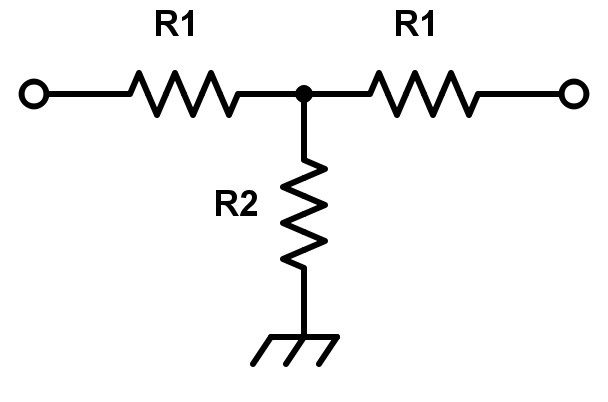## This calculator helps you determine the values of the resistors R1 and R2 to be used for a T-pad attenuator.

(dB)
(Ω)

(Ω)
(Ω)

### Overview

This calculator is designed to help calculate the value of the resistors R1 and R2 to be used in T-pad attenuator. The user only needs to enter the attenuation in decibels (dB) and the given characteristic impedance of the transmission lines to be matched.### Equation

$$R_{1}=Z_{0}\left [ \frac{10^{\frac{A_{dB}}{20}}-1}{10^{\frac{A_{dB}}{20}}+1}\right ]$$

$$R_{2}=2Z_{0}\left [ \frac{10^{\frac{A_{dB}}{20}}}{10^{\frac{A_{dB}}{10}}-1}\right ]$$

Where:

$$R_{1}$$ = T-pad attenuator resistor 1

$$R_{2}$$ = T-pad attenuator resistor 2

$$Z_{0}$$ = transmission line characteristic impedance

$$A_{dB}$$ = desired attenuation in dB

### Applications

An attenuator is a passive network, designed to weaken the power being supplied from a source circuit to a level suitable to the destination circuit. The theory behind attenuators comes from the voltage divider principle.

In RF applications, it is normally desired to use an attenuator to reduce signal levels from one point in the network to the other. Besides this, attenuators are also used to match impedances between two circuits in order for maximum power transference. Attenuators can also be used to isolate two different circuit stages.

The T-pad attenuator is one of the more common passive attenuators used in RF applications. The advantage of this network over other topologies is its simple construction. It is easier to etch out a T-pad network on a thin film circuit compared to etching a balanced or bridged-tee attenuator circuit.

Bridged-Tee Attenuator Calculator

Balanced Attenuator Calculator

Pi Attenuator Calculator

Reflection Attenuator Calculator

Textbook - Attenuators: Amplifiers and Active Devices

Textbook - Divider Circuits and Kirchoff's Laws

Textbook - Impedance Transformations•Hieu Nguyen 1 July 19, 2018
•RK37 July 20, 2018